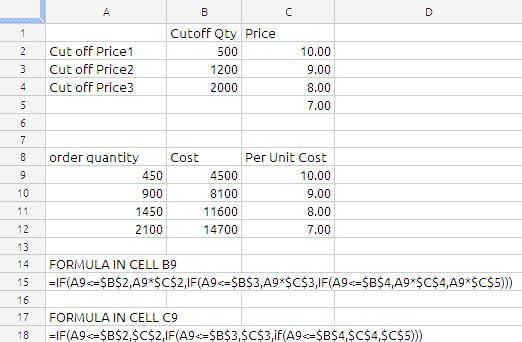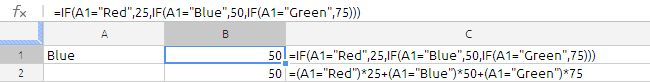## IF FUNCTION

The IF function returns one of two values depending on whether a specified expression evaluates to a Boolean value of TRUE or FALSE.

IF(test, then_value, otherwise_value)

test:  A logical expression. test can contain anything as long as the expression can be evaluated as a Boolean. If the expression evaluates to a number, 0 is considered to be FALSE, and any other number is considered to be TRUE.

then_value:  The value returned if the expression is TRUE. if-true can contain any value type. If omitted (comma but no value), IF will return 0.

otherwise_value:  An optional argument specifying the value returned if the expression is FALSE. if-false can contain any value type. If omitted (comma but no value), IF will return 0. If entirely omitted (no comma after if-false) and if-expression evaluates to FALSE, IF will return FALSE.

Usage Notes

(1) If the Boolean value of test is TRUE, the function returns the then_value; otherwise it returns the otherwise_value. Both then_value and otherwise_value can contain additional IF functions (nested IF functions).

(2) the then_value & otherwise_value is optional argument. if left it still the IF function works. if the test results in 1/0 or TRUE/FALSE the result displayed by the function is TRUE/FALSE depending on the test value evaluated.

What is Boolean:

A Boolean value is one that can be expressed in only one of two values, as either TRUE or as FALSE. A FALSE value also has the value of 0. In this case, any other numeric value is considered TRUE. Boolean values are mostly used in comparisons.

Lets understand the IF function with an examples

Exp:1

If i order up to 500 units of the a product, I pay 10.00 per unit. if order from 501 through 1200 units, i pay 9.00 per unit. if i order from 1201 through 2000 units, i pay 8 per unit. if i order more than 2000 units, i pay 7 per unit. How can i write formula that expresses the purchase cost as a function of the number of units purchased?we have used the nested IF conditions as shown in above image.

Exp 2:

If the value in Cell A1 is Red than value in the cell B1 will be 25 , if it is Blue then B1 has to be 50 and if A1 is Green then value in B1 has to be 75.

Solution:in this case we have used two methods using IF function and using boolean algebra, Some time this type of situation using of boolean algebra will much more understandable and it is not constrained by IF function limitations.

This simple comparison will result in a value of TRUE if A2 has “Red” in it (just to be clear: the string of letters, not the background color). If there is anything else or even nothing in A2, then the result will be FALSE. True and False are actual values in the spreadsheet. If you were to enter this formula in some cell, you would see the word TRUE or the word FALSE in that cell, depending on what was in A2.

the spreadsheet is evaluated as shown below:

=(False)*25 + (True)*50 + (False)*75

= (0)*25+(1)*50+(0)*75

=0+50+0

=50

the other method of evaluating this is by use of CHOOSE function, the formula will be

=choose(MATCH(A1,F1:F3,0),25,50,75)

where F1 to F3 will hold Red,Blue, Green

we can also use the vlookup

The IF function has endless uses so we cannot document everything in one article. so i am concluding this article.